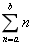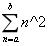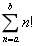# 浅谈函数式编程

1. 函数式编程使用不可变对象作为变量，不会修改变量的值，而是返回一个新的值，如此这样，更容易理清头绪，使得单元测试和调试更加容易；
2. 可以很自由地传递不可变对象，但对于可变对象来说，传递给其他代码之前，需要先建造一个以防万一的副本；
3. 一旦不可变对象完成构造以后，就不会有线程因为并发访问而破坏对象内部状态，因为根本没有线程可以改变不可变对象的状态；
4. 不可变对象让哈希表键值更安全，所以哈希表键要求必须是不可变对象，否则使用可变对象，如果对象状态发生变化，那么在哈希表中就找不到这个对象了；

Java函数式编程举例：

 1 package lxy.java.fp;
2
3 import java.util.*;
4 import java.util.function.*;
5
6
7 public class FPDemo {
8     //定义泛型方法，用以根据第二个参数指定的条件从第一个参数指定的集合中过滤部分元素，并返回过滤后的结果。这里的第二个参数是一个函数式接口。
9     public static <T> List <T> filter(List <T> list, Predicate <T> p) {
10         List <T> results = new ArrayList <>();
11         for (T s : list) {
12             if (p.test(s)) {
14             }
15         }
16         return results;
17     }
18
19     public static void main(String[] args) {
20         List <String> myList = Arrays.asList("Hello", "Java", "Python", "Scala");
21
22         //通过匿名类的方式
23         List <String> results = filter(myList, new Predicate <String>() {
24             public boolean test(String t) {
25                 return t.length() >= 5;
26             }
27         });
28         System.out.println("through anonymous class:");
29         System.out.println("strings with length more than 5:");
30         for (String result : results) {
31             System.out.println(result);
32         }
33
34         //行为参数化，通过匿名函数（即lambda表达式）方式，
35         System.out.println("through lambda expression:");
36         System.out.println("strings with length more than 5:");
37         List <String> results2 = filter(myList, s -> s.length() >= 5);
38         results2.forEach(s -> System.out.println(s));
39
40         //很容易地将过滤条件由字符串长度大于等于5改为字符串以字母a结尾，
41         // 这就是行为参数化，即将具体的逻辑（即行为或者函数）参数化，使得filter函数更加抽象，提高了代码复用度
42         //否则需要写2个filter函数，一个过滤出长度大于等于5的字符串，另一个过滤出以字符a结尾的字符串
43         System.out.println("strings ends with character 'a'");
44         List <String> results3 = filter(myList, s -> s.endsWith("a"));
45         results3.forEach(System.out::println);
46
47         //Java流中的filter函数和map函数，注意这里的filter是Java库函数，和前面自定义的filter函数不一样
48         System.out.println("strings with length more than 5 and its length:");
49         myList.parallelStream().filter(s -> s.length() >= 5).map(s -> "(" + s + ", " + s.length() + ")")
50                 .forEach(System.out::println);
51
52         //高阶函数
53         Function <Integer, Integer> f = (Integer x) -> x + 1;
54         Function <Integer, Integer> g = (Integer x) -> x * x;
55         Function <Integer, Integer> h = f.andThen(g);
56         Function <Integer, Integer> r = f.compose(g);
57
58         System.out.println("higher-order function:");
59         System.out.println("h(2)=g(f(2))=" + h.apply(2));
60         System.out.println("r(2)=f(g(2))=" + r.apply(2));
61
62     }
63 }

Scala函数式编程举例：

 1 package lxy.scala.fp
2
3 object FPDemo {
4     //定义泛型方法，用以根据第二个参数指定的条件从第一个参数指定的列表中过滤部分元素，并返回过滤后的结果，结果类型仍然是List。
5     //这里的第二个参数是一个函数, 该函数输入参数类型为T，返回值类型为Boolean
6     def filter[T](list: List[T], f: T => Boolean) =
7         for {e <- list if f(e) == true} yield e
8
9     //高阶函数，该函数定义为g(f(x))，其中函数f和g都是作为参数在调用该高阶函数时指定的
10     def highOrderFunction1(x: Int, f: Int => Int, g: Int => Int) = g(f(x))
11
12     //定义嵌套函数，针对每个参数，外层函数都会返回一个函数，即内层函数
13     //这里factor是自由变量，number是绑定变量。
14     //闭包是一个函数，返回值依赖于声明在函数外部的一个或多个变量
15     //函数multiplier返回的函数就是闭包，factor就是外部的变量，也叫自由变量，number是绑定变量(形式参数）
16     def multiplier(factor: Int): Int => Int = {
17         def multiplyByFactor(number: Int) = factor * number
18
19         return multiplyByFactor
20     }
21
22     //柯里化函数，类型是(Int)(Int) => Int
23     def multiplier2(factor: Int)(number: Int) = factor * number
24
25     //这个函数实际跟multiplier效果是一样的，每传入一个参数factor，都会返回一个函数
26     //闭包是一个函数，返回值依赖于声明在函数外部的一个或多个变量
27     //函数multiplier3返回的函数就是闭包，factor就是外部的变量，也叫自由变量，number是绑定变量(形式参数）
28     def multiplier3(factor: Int) = multiplier2(factor) _
29
30     def main(args: Array[String]): Unit = {
31         val myList = List("Hello", "Java", "Python", "Scala")
32         println("strings with length more than 5")
33         filter(myList, (s: String) => s.length >= 5).foreach(s => println(s))
34
35         println("strings ends with character 'a'")
36         filter(myList, (s: String) => s.endsWith("a")).foreach(println)
37
38         println("strings with length more than 5 and its length:")
39         //这里的filter不是自定义函数filter，而是库函数，返回长度大于等于5的字符串以及对应的长度
40         myList.filter(_.length >= 5).map(s => (s, s.length)).foreach(println)
41
42
43         val result = highOrderFunction1(2, (x: Int) => x + 1, (x: Int) => x * x)
44         println("f(x)=x+1, g(x)=x*x, g(f(2))=" + result)
45
46
47         println("multiplier(2)=" + multiplier(2))
48         println("multiplier(2)(3)=" + multiplier(2)(3))
49         val double = multiplier(2)
50         println("double(3)=" + double(3))
51
52         println("multiplier3(3)=" + multiplier3(3))
53         println("multiplier3(3)(4)=" + multiplier3(3)(4))
54         val triple = multiplier3(3)
55         println("triple(4)=" + triple(4))
56
57     }
58 }

Python函数式编程举例：

 1 #!/usr/bin/env python3
2 # -*- coding: utf-8 -*-
3
4 if __name__ == "__main__":
5     """
6         Usage: ./fp_demo.py
7     """
8
9     # 定义函数filter，第一个参数是列表，第二个参数是函数
10     def filter(list, f):
11         return [e for e in list if f(e) == True]
12
13     # python中没有foreach函数，自己定义一个，其中第一个参数是函数，第二个参数是迭代器（列表）
14     def foreach(f, iterator):
15         for item in iterator:
16             f(item)
17
18     myList = ["Hello", "Java", "Python", "Scala"]
19     resultList = filter(myList, lambda e: len(e) >= 5)
20     print("strings with length more than 5", sep="\n")
21     foreach(lambda e: print(e, sep="\n"), resultList)
22
23     resultList2 = filter(myList, lambda e: e.endswith("a"))
24     print("strings ends with character 'a'", sep="\n")
25     foreach(lambda e: print(e, sep="\n"), resultList2)
26
27     # 这里的map函数是python内置的
28     print("strings with length more than 5 and its length:", sep="\n")
29     foreach(lambda e: print(e, sep="\n"), map(lambda e: (e, len(e)), filter(myList, lambda e: len(e) >= 5)))
30
31     # 高阶函数，该函数定义为g(f(x))，其中函数f和g都是作为参数在调用该高阶函数时指定的
32     def highOrderFunction1(x, f, g):
33         return g(f(x))
34
35     # 该函数在下面对highOrderFunction1函数的调用中被当做参数传入
36     def f(x):
37         return x + 1
38
39     # 第二个参数传入上面定义的f函数，作为第三个参数的的函数采用的是匿名函数
40     result = highOrderFunction1(2, f, g=lambda x: x * x)
41     print("f(x)=x+1, g(x)=x*x, g(f(2))=%d" % result, sep="\n")
42
43     # 定义嵌套函数，针对每个参数，外层函数都会返回一个函数，即内层函数
44     # 这里factor是自由变量，number是绑定变量。
45     # 闭包是一个函数，返回值依赖于声明在函数外部的一个或多个变量
46     # 函数multiplier返回的函数就是闭包，factor就是外部的变量，也叫自由变量，number是绑定变量(形式参数）
47     def multiplier(factor):
48         def multiplyByFactor(number):
49             return factor * number
50         return multiplyByFactor
51
52
53     print("multiplier(2)(3)=%s" % multiplier(2)(3))
54     # double是一个函数，它将输入参数乘以2倍以后返回
55     double = multiplier(2)
56     print("double(3)=%s" % double(3))
57
58     print("multiplier(3)(4)=%s" % multiplier(3)(4))
59     # triple是一个函数，它将输入参数乘以3倍以后返回
60     triple = multiplier(3)
61     print("triple(4)=%s" % triple(4))
62
63     # 第三个参数f是函数
65         return f(x) + f(y)
66
67     print("2 ^ 2 + 3 ^2 = %s" % add(2, 3, lambda x: x * x))
68     print("(2 + 1) + (3 + 1) = %s" % add(2, 3, f))可以看到这三个公式分别是求a~b的和，a~b的平方和，a~b各自的阶乘的和。可以看到三个公式是类似的，都是求和，只不过分别是对自身求和，对自身的平方求和以及对自身的阶乘求和，也就是说这里有3个计算逻辑，需要对这3个计算逻辑计算出来的数求和。如果是指令式编程风格，就只能写三个函数来解决问题。但是如果采用函数式编程风格，就可以只写一个通用的求和函数来解决该问题，因为可以将这3个计算逻辑（函数）作为参数传给之前的通用求和函数。下面分别用Java,ScalaPython来解决该问题。

Java代码

 1 package lxy.java.fp;
2
3 import java.util.function.*;
4
5
6 public class Sum4Integers {
7     //通用求和函数，其中f是一个函数式接口，它接收一个整型参数并返回一个整型数值
8     //采用递归计算方法
9     private static int sum(Function<Integer, Integer> f, int a, int b) {
10         if (a > b)
11             return 0;
12         else
13             return f.apply(a) + sum(f, a + 1, b);
14     }
15
16     //求阶乘函数,采用递归算法，较复杂，不能作为匿名函数传入上面的通用求和参数，因此需要预先定义
17     private static int factor(int x) {
18         if (x == 0)
19             return 1;
20         else
21             return x * factor(x - 1);
22     }
23
24     //求公式一函数，即求a~b的和
25     //作为参数的函数就是返回变量自身，较简单，采用匿名函数
26     static int sumInts(int a, int b) {
27         return sum(x -> x, a, b);
28     }
29
30     //求公式二函数，即求a^2 ~ b^2的和
31     //作为参数的函数就是返回变量的平方，较简单，采用匿名函数
32     static int sumSquares(int a, int b) {
33         return sum(x -> x * x, a, b);
34     }
35
36     //求公式三函数，即求a! ~ b!的和
37     //作为参数的函数就是求变量的阶乘，较复杂（本身是递归函数），采用定义好的函数factor
38     static int sumFactors(int a, int b) {
39         return sum(Sum4Integers::factor, a, b);
40     }
41
42     public static void main(String[] args) {
43         System.out.println("1+2+3+4+5=" + sumInts(1, 5));
44         System.out.println("1^2+2^2+3^2+4^2+5^2=" + sumSquares(1, 5));
45         System.out.println("1!+2!+3!+4!+5!=" + sumFactors(1, 5));
46     }
47
48 }

Scala代码

 1 package lxy.scala.fp
2
3
4 object Sum4Integers {
5     //通用求和函数
6     //采用递归计算方法
7     private def sum(f: Int => Int, a: Int, b: Int): Int = if (a > b) 0 else f(a) + sum(f, a + 1, b)
8
9     //求阶乘函数,采用递归算法，较复杂，不能作为匿名函数传入上面的通用求和参数，因此需要预先定义
10     private def factor(x: Int): Int = if (x == 0) 1 else x * factor(x - 1)
11
12     //求公式一函数，即求a~b的和
13     //作为参数的函数就是返回变量自身，较简单，采用匿名函数
14     def sumInts(a: Int, b: Int) = sum(x => x, a, b)
15
16     //求公式二函数，即求a^2 ~ b^2的和
17     //作为参数的函数就是返回变量的平方，较简单，采用匿名函数
18     def sumSquares(a: Int, b: Int) = sum(x => x * x, a, b)
19
20     //求公式三函数，即求a! ~ b!的和
21     //作为参数的函数就是求变量的阶乘，较复杂（本身是递归函数），采用定义好的函数factor
22     def sumFactors(a: Int, b: Int) = sum(factor, a, b)
23
24     def main(args: Array[String]): Unit = {
25         println("1+2+3+4+5=" + sumInts(1, 5))
26         println("1^2+2^2+3^2+4^2+5^2=" + sumSquares(1, 5))
27         println("1!+2!+3!+4!+5!=" + sumFactors(1, 5))
28     }
29
30 }

Python代码

 1 #!/usr/bin/env python3
2 # -*- coding: utf-8 -*-
3
4 if __name__ == "__main__":
5     """
6         Usage: ./sum_integers.py
7     """
8
9     # 通用求和函数，采用递归计算方法
10     def sum(f, a, b):
11         if a > b:
12             return 0
13         else:
14             return f(a) + sum(f, a + 1, b)
15
16     # 求阶乘函数,采用递归算法，较复杂，不能作为匿名函数传入上面的通用求和参数，因此需要预先定义
17     def factor(x):
18         if x == 0:
19             return 1
20         else:
21             return x * factor(x - 1)
22
23     # 求公式一函数，即求a~b的和
24     # 作为sum函数的第一个参数的函数就是返回变量自身，较简单，采用匿名函数
25     def sumInts(a, b):
26         return sum(lambda x: x, a, b)
27
28     # 求公式二函数，即求a^2 ~ b^2的和
29     # 作为sum函数的第一个参数的函数就是返回变量的平方，较简单，采用匿名函数
30     def sumSquares(a, b):
31         return sum(lambda x: x * x, a, b)
32
33     # 求公式三函数，即求a! ~ b!的和
34     # 作为sum函数的第一个参数的函数就是求变量的阶乘，较复杂（本身是递归函数），采用定义好的函数factor
35     def sumFactors(a, b):
36         return sum(factor, a, b)
37
38     print("1+2+3+4+5=%d" % sumInts(1, 5))
39     print("1^2+2^2+3^2+4^2+5^2=%d" % sumSquares(1, 5))
40     print("1!+2!+3!+4!+5!=%d" % sumFactors(1, 5))

posted @ 2019-12-04 20:45  白竹山  阅读(...)  评论(...编辑  收藏